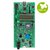## I am trying to pass an integer parameter to the lcd and i am getting an error message "3/155"

1. include "mbed.h"
2. include "LCD_DISCO_L476VG.h"
3. include "TextLCD.h"

LCD_DISCO_L476VG lcd; DigitalIn countup(PA_0); DigitalIn countdown(PA_5); DigitalOut greenled(LED1); DigitalOut redled(LED2); InterruptIn button(USER_BUTTON);

int i=16;

void pressed(){ greenled=1; redled=0; i=0; }

int x={0, 1, 2, 3, 4, 5, 6, 7, 8, 9, 10, 11, 12, 13, 14, 15};

int main() { uint8_t title[] = {" PARKING LOT COUNTER"}; uint8_t spots[] = {" REMAING SPOTS"}; uint8_t start[] = {"15/15"}; uint8_t fifthteen[] = {"14/15"}; uint8_t fourteen[] = {"13/15"}; uint8_t thirteen[] = {"12/15"}; uint8_t twelve[] = {"11/15"}; uint8_t eleven[] = {"10/15"}; uint8_t ten[] = {"9/15"}; uint8_t nine[] = {"8/15"}; uint8_t eight[] = {"7/15"}; uint8_t seven[] = {"6/15"}; uint8_t six[] = {"5/15"}; uint8_t five[] = {"4/15"}; uint8_t four[] = {"3/15"}; uint8_t three[] = {"2/15"}; uint8_t two[] = {"1/15"}; uint8_t one[] = {"0/15"};

printf("\nPARKING LOT COUNTER\n"); lcd.ScrollSentence(title, 1, 700); greenled = 1; lcd.Clear(); lcd.ScrollSentence(spots, 1, 700); wait(1.0); redled = 0;

lcd.Clear(); lcd.DisplayString(start);

button.rise(&pressed);

while(1) { if(countdown==1) i; if(countup==1) i++; if(i==0){ greenled=0; redled=1; } else{ greenled=1; redled=0; } if(i==16) lcd.DisplayString(start); if(i==15) lcd.DisplayString(fifthteen); if(i==14) lcd.DisplayString(fourteen); if(i==13) lcd.DisplayString(thirteen); if(i==12) lcd.DisplayString(twelve); if(i==11) lcd.DisplayString(eleven); if(i==10) lcd.DisplayString(ten); if(i==9) lcd.DisplayString(nine); if(i==8) lcd.DisplayString(eight); if(i==7) lcd.DisplayString(seven); if(i==6) lcd.DisplayString(six); if(i==5) lcd.DisplayString(five); if(i==4) lcd.DisplayString(four); if(i==3) lcd.DisplayString(three); if(i==2) lcd.DisplayString(two); if(i==1) lcd.DisplayString(one);

} }

#### Question relating to:The 32L476GDISCOVERY helps users to develop and share applications with the STM32L4 ultra-low-power microcontrollers.

```<<code>>
<</code>>
```

And when you say you get that error can you be more specific: When you run the code, when you compile the code? If it's a compile error does it give a line number?

posted by Andy A 21 Nov 2019
Comment on this question